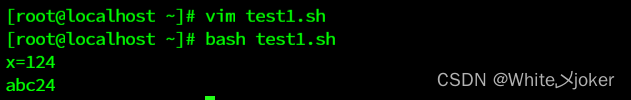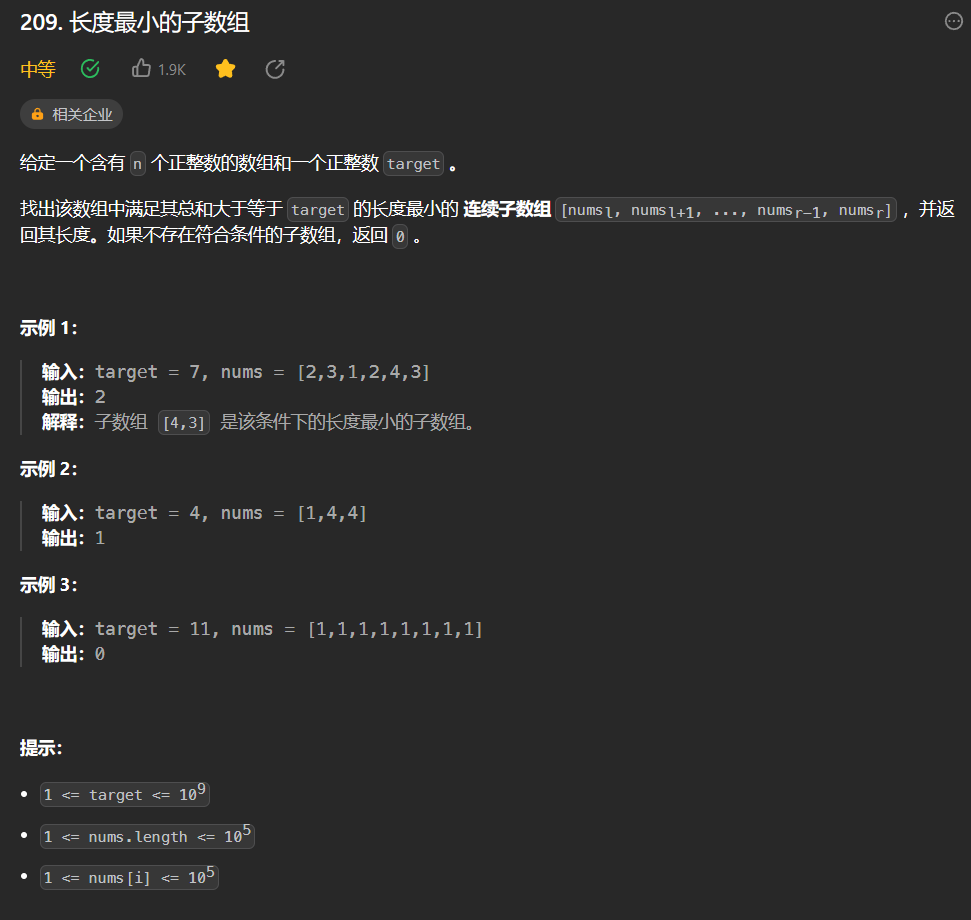# 【深度学习】分类损失函数解析

## 【深度学习】分类相关的损失解析

### 文章目录

• 【深度学习】分类相关的损失解析
• 1. 介绍
• 2. 解析
• 3. 代码示例

## 1. 介绍## 2. 解析

• 二元交叉熵损失（Binary Cross Entropy Loss，BCELoss）：
torch.nn.BCELoss() 是用于二元分类的损失函数。它将模型输出的概率与真实标签的二进制值进行比较，并计算二元交叉熵损失。BCELoss 可以处理每个样本属于多个类别的情况。当使用 BCELoss 时，需要注意模型输出经过 sigmoid 激活函数转换为 [0, 1] 的概率形式。

• 带 logits 的二元交叉熵损失（Binary Cross Entropy With Logits Loss，BCEWithLogitsLoss）：
torch.nn.BCEWithLogitsLoss() 是和 BCELoss 相似的损失函数，它同时应用了 sigmoid 函数和二元交叉熵损失。在使用 BCEWithLogitsLoss 时，不需要对模型输出手动应用 sigmoid 函数，因为该函数内部已经自动执行了这个操作。

• 多类别交叉熵损失（Multiclass Cross Entropy Loss，CrossEntropyLoss）：
torch.nn.CrossEntropyLoss() 是用于多类别分类任务的损失函数。它将模型输出的每个类别的分数与真实标签进行比较，并计算交叉熵损失。CrossEntropyLoss 适用于每个样本只能属于一个类别的情况。注意，在使用 CrossEntropyLoss 前，通常需要确保模型输出经过 softmax 或 log softmax 函数。

• 多标签二元交叉熵损失（Multilabel Binary Cross Entropy Loss）：
当每个样本可以属于多个类别时，我们可以使用二元交叉熵损失来处理多标签分类任务。对于每个样本，将模型输出的概率与真实标签进行比较，并计算每个标签的二元交叉熵损失。可以逐标签地对每个标签应用 BCELoss，或者使用 torch.nn.BCEWithLogitsLoss() 并将模型输出中的最后一个维度设置为标签数量。文章来源地址:https://www.uudwc.com/A/oLxkp/

## 3. 代码示例

1）二元交叉熵损失（BCELoss）：

import torch
import torch.nn as nn

# 模型输出经过 sigmoid 函数处理
model_output = torch.sigmoid(model(input))
# 真实标签
target = torch.Tensor([0, 1, 1, 0])
# 创建损失函数对象
loss_fn = nn.BCELoss()
# 计算损失
loss = loss_fn(model_output, target)


2）带 logits 的二元交叉熵损失（BCEWithLogitsLoss）：

import torch
import torch.nn as nn

# 模型输出未经过 sigmoid 函数处理
model_output = model(input)
# 真实标签
target = torch.Tensor([0, 1, 1, 0])
# 创建损失函数对象
loss_fn = nn.BCEWithLogitsLoss()
# 计算损失
loss = loss_fn(model_output, target)


3）多类别交叉熵损失（CrossEntropyLoss）：

import torch
import torch.nn as nn

# 模型输出经过 softmax 函数处理
model_output = nn.functional.softmax(model(input), dim=1)
# 真实标签（每个样本只能属于一个类别）
target = torch.LongTensor([2, 1, 0])
# 创建损失函数对象
loss_fn = nn.CrossEntropyLoss()
# 计算损失
loss = loss_fn(model_output, target)


4）多标签二元交叉熵损失（Multilabel Binary Cross Entropy Loss）：

import torch
import torch.nn as nn

# 模型输出未经过 sigmoid 函数处理
model_output = model(input)
# 真实标签
target = torch.Tensor([[0, 1], [1, 1], [1, 0], [0, 1]])
# 创建损失函数对象
loss_fn = nn.BCEWithLogitsLoss()
# 计算损失，将模型输出的最后一个维度设置为标签数量
loss = loss_fn(model_output, target)